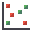The Rocscience International Conference 2021 Proceedings are now available. Read Now

# Scatter Plot

To create a Scatter Plot after a Probabilistic Analysis:

1. Select Plot Scatterfrom the toolbar or the Statistics menu.
2. Select the data you would like to plot on the X-axis and Y-axis (X Axis Dataset, and Y Axis Data Set).
3. When it is relevant, select a Location:
• Single Segment: Select the specific segment you would like to plot from the drop-down menu.
• Multiple Segments: Select the segment(s) you would like to plot. Click Pick Segments and right-click and drag the mouse to select the segments you are interested in. Press ENTER.
• End Wedges: Select the wedge you would like to plot from the drop-down menu..
4. The display of the regression line may be toggled on or off with the Show Regression Line checkbox.
5. Select OK.

The Scatter Plot will be generated. If your regression line is on, you will see a listing of Correlation Coefficient, Alpha and Beta, at the bottom of the Scatter Plot.

Correlation Coefficient

The Correlation Coefficient indicates the degree of correlation between the two variables plotted. The Correlation Coefficient can vary between -1 and 1 where numbers close to zero indicate a poor correlation, and numbers close to 1 or -1 indicate a good correlation. Note that a negative correlation coefficient simply means that the slope of the best fit linear regression line is negative.

Alpha and Beta

Alpha and beta represent the y-intercept and slope, respectively, of the best fit linear regression line to the scatter plot data. The linear regression line can be displayed on the plot with the Show Regression Line option.

Right-click Options

The following options are available in the right-click menu for Scatter Plots: